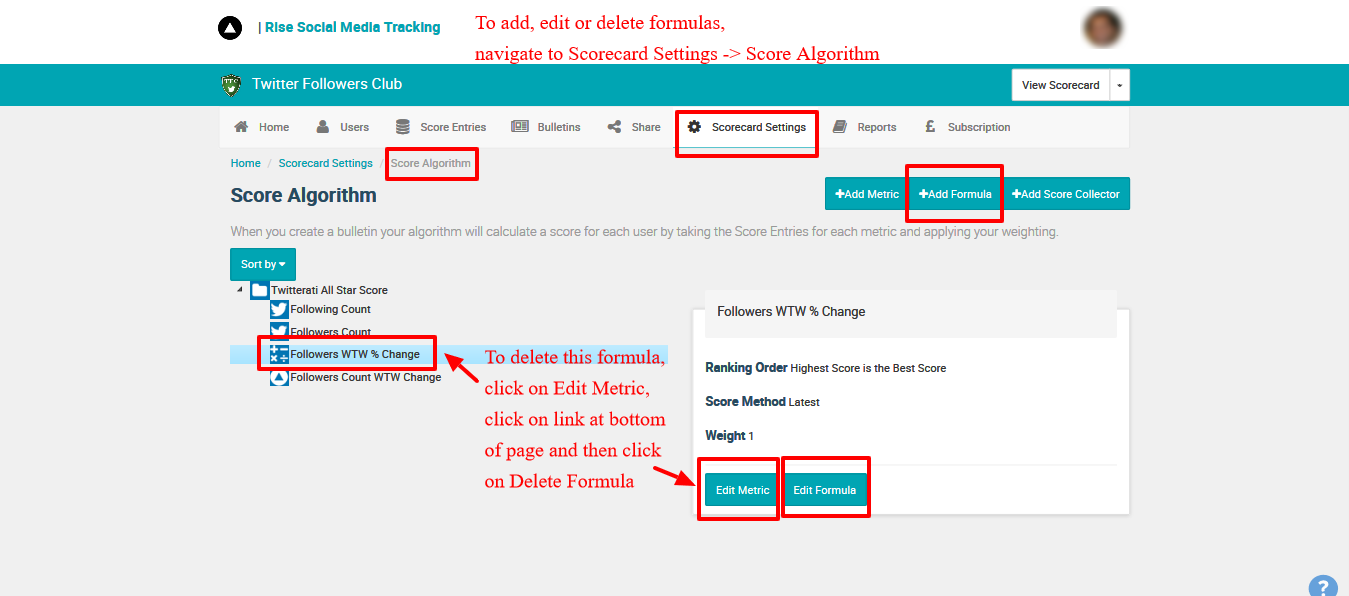Formulas allow you to produce more complex metrics such as ratios (RTs per 100 tweets for example) or (% target achieved).

1. Go to scorebook settings -> score algorithm.
2. Click on the +Add Formula button
3. This will then take you to the screen to add the Formulas page, where you can enter a Formula. This is made up of calculator style operators (+ - / * ) and brackets. You can use any other metric as an input into your formula.
4. To edit Formulas once created, you do Step 1 and then highlight the Formula by clicking on its name within the Metric Tree, and then click on Edit Formula
5. To delete a Formula from your score algorithm, repeat Step 4, but instead of Edit Formula, click on Edit Metric, go to the bottom of the page, click on the link which says "This metric is derived from xxxx" and on the resulting page, click on the red button "Delete Formula"Note that you can use Custom Fields to store static variables and ranges.

# Available Functions

Rise Formulas adhere to the open formula standard as closely as possible.

## ABS

Summary: Gives the positive version of a number

Syntax: ABS( Number X )

## IFNEG

Summary: Returns to value if the number is negative

Syntax: IFNEG( Number X, Value )

## IFPOS

Summary: Returns to value if the number is positive

Syntax: IFPOS( Number X, Value )

## IFZERO

Summary: Returns to value if the number is zero

Syntax: IFZERO( Number X ; Value )

## LOG10

Summary: Return the base 10 logarithm of a number.

Syntax: LOG10( Number N )

## MAX

Summary: Returns  the highest of two numbers

Syntax: MAX( Number X ; Number Y )

## MIN

Summary: Returns  the lowest of two numbers

Syntax: MIN( Number X ; Number Y )

## RLOOKUP

Summary: Returns  the value from a range based on a number

Syntax: RLOOKUP( Number X ; Range )

A range takes the format:  {threshold,value;threshold,value} i.e. {99,1;199,2;299,3} would return 1 for values <=99, 2 for values 100-199 and 3 for values 200-299.

RLOOKUP will return error codes on incorrect formatting as follows:

-9991 = No lookup pair found - minimum format should be { threshold , result }

-9992 = A lookup pair is incorrectly specified. i.e. it is missing its comma - format should be  { threshold , result ; threshold , result }, you have an extra ; at the end of your lookup list

-9993 = A lookup pair has more than two parts. i.e. you have an extra comma

0 = no lookup pair could be found that matched the value

## ROUND

Summary: Rounds the value X to the nearest multiple of the power of 10 specified by Digits.

Syntax: ROUND( Number X [ ; Number Digits = 0 ] )

## ROUNDDOWN

Summary: Rounds the value X towards zero to the number of digits specified by Digits

Syntax: ROUNDDOWN( Number X [ ; Integer Digits = 0 ] )

ROUNDUP

Summary: Rounds the value X away from zero to the number of digits specified by Digits

Syntax: ROUNDUP( Number X [ ; Integer Digits = 0 ] )